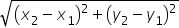Maths-
General
Easy

Question

# Find the distance between (-1,4) and (3 ,7)

## The correct answer is: 5 units

### On comparing (-1,4) and (3,7) with coordinates points (x1,y1 ) and (x2,y2 ) respectively we have,x1=-1;   y1=4x2=3;    y2=4Distance between two points= 5 units#### With Turito Foundation.#### Get an Expert Advice From Turito.